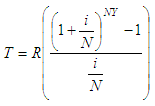# Calculate Annuity - or Future Value of Regular Deposits

In this article we create a script to calculate annuity or to find out the future value of a regular deposit.

 You must provide the amount of each deposit, the number of deposits per year, the number of years and the nominal interest rate. Assuming that interest is compounded with each deposit, the calculation is based on the following formula:where:
T = total value after Y years (future value)
R = amount of regular deposits
N = number of deposits per year
Y = number of years
i = nominal interest rate

This presentation shows you what we want to accomplish with an example. Below the presentation, I give you the full code to implement in Matlab.

This is a suggested Matlab function or script to calculate the future-value formula above. You have four inputs and one output:

function t = annuity(r, it, n, y)
it = it/(n*100);
t = r * ((1 + it)^(n * y) - 1)/it;

We can create another script to test and run the above m-file:

clc; clear; format bank

r = input('Enter amount of regular deposits: ');
it = input(
'Enter nominal interest rate: ');
n = input(
'Enter number of deposits per year: ');
y = input(
'Enter number of years: ');

future_value = annuity(r, it, n, y)

Example 1:

\$50 is transferred each month from Monique’s checking account to a Math Learning Club savings account with 5% interest. How much will Monique receive at the end of the year?

We run our test code and get:

Enter amount of regular deposits: 50
Enter nominal interest rate: 5
Enter number of deposits per year: 12
Enter number of years: 1

Our result is:

future_value = 613.94

Example 2
:

Little George makes annuity payments of \$175. The interest is 5.5%. What amount will George have accumulated in 15 years?

Again, we run our ‘Calculate Annuity’ code, and get:

Enter amount of regular deposits: 175
Enter nominal interest rate: 5.5
Enter number of deposits per year: 1
Enter number of years: 15

The result is:

future_value = 3921.5

From 'Calculate Annuity' to home

From 'Calculate Annuity' to 'Finance Formulas'